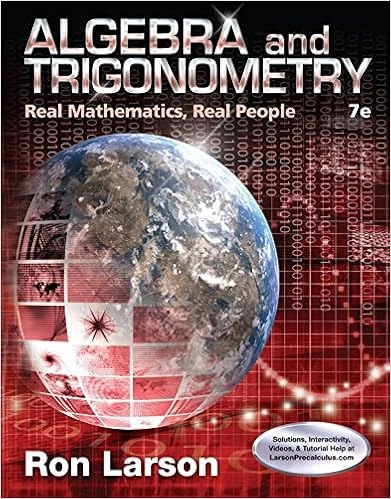# Solids with holes the previous ideas and techniques

• Homework Help
• 89
• 71% (14) 10 out of 14 people found this document helpful

This preview shows page 8 - 12 out of 89 pages.

##### We have textbook solutions for you!
The document you are viewing contains questions related to this textbook.The document you are viewing contains questions related to this textbook.
Chapter 7 / Exercise 56
Algebra and Trigonometry: Real Mathematics, Real People
LarsonExpert Verified
Solids With Holes The previous ideas and techniques can also be used to find the volumes of solids with holes in them. If A(x) is the area of the face formed by a cut at x , then it is still true that the volume is V = a b A( x ) dx . However, if the solid has holes, then some of the faces will also have holes and a formula for A( x ) may be more complicated.
##### We have textbook solutions for you!
The document you are viewing contains questions related to this textbook.The document you are viewing contains questions related to this textbook.
Chapter 7 / Exercise 56
Algebra and Trigonometry: Real Mathematics, Real People
LarsonExpert Verified
5.1 Volumes Contemporary Calculus 9 Sometimes it is easier to work with two integrals and then subtract: (i) calculate the volume S of the solid without the hole, (ii) calculate the volume H of the hole, and (iii) subtract H from S. Example 10 : Calculate the volume of the solid in Fig. 26. Solution: The face for a slice at x , has area A( x ) = { area of large circle } { area of small circle } = π { large radius } 2 π { small radius } 2 = π { x + 1 } 2 π { 1/ x } 2 = π ( x 2 + 2 x + 1 – 1/ x 2 ). Then Volume = a b A( x ) dx = 1 2 π ( x 2 + 2 x + 1 – 1/ x 2 ) dx = π { 1 3 x 3 + x 2 + x + 1/ x } | 2 1 18.33 . Alternately, the volume of the solid with the large circular faces is 1 2 π ( x 2 + 2 x + 1 ) dx = 19 π 3 19.90 , and the volume of the hole is 1 2 π ( 1/ x 2 ) dx = π 2 1.57 so the volume we want is 19.90 – 1.57 = 18.33 . Practice 7 : Calculate the volume of the solid in Fig. 27 . WRAP UP At first, all of these volumes may seem overwhelming –– there are so many possible solids and formulas and different cases. If you concentrate on the differences, it is very complicated. Instead, focus on the pattern of cutting, finding areas of faces, volumes of slices, and adding . With that pattern firmly in mind, you can reason your way to the definite integral. Try to make cuts so the resulting faces have regular shapes (rectangles, triangles, circles) whose areas you can calculate. Try not to let the complexity of the whole solid confuse you. Sketch the shape of one face and label its dimensions. If you can find the area of one face in the middle of the solid, you can usually find the pattern for all of the faces and then you can easily set up the integral.
5.1 Volumes Contemporary Calculus 10 PROBLEMS In problems 1 – 6, use the values given in the tables to calculate the volumes of the solids. (Fig. 28 – 33) Table 1: box base height thickness Table 2: box base height thickness (Fig. 28) 1 5 6 1 (Fig. 29) 1 5 6 2 2 4 4 2 2 5 4 1 3 3 3 1 3 3 3 1 4 2 2 1 Table 3: disk radius thickness Table 4: disk height thickness (Fig. 30) 1 4 0.5 (Fig. 31) 1 8 0.5 2 3 1 2 6 1 3 1 2 3 2 2 Table 5: slice face area thickness Table 6: slice rock area min. area thickness (Fig. 32) 1 9 0.2 (Fig. 33) 1 4 1 0.6 2 6 0.2 2 12 1 0.6 3 2 0.2 3 20 4 0.6 4 10 3 0.6 5 8 2 0.6 3 Fig. 28 1 2
5.1 Volumes Contemporary Calculus 11 In problems 7 – 12, represent each volume as an integral and evaluate the integral. 7. Fig. 34. For 0 x 3, each face is a rectangle with base 2 inches and height 5– x inches. 8. Fig. 35. For 0 x 3, each face is a rectangle with base x inches and height x 2 inches.
•••